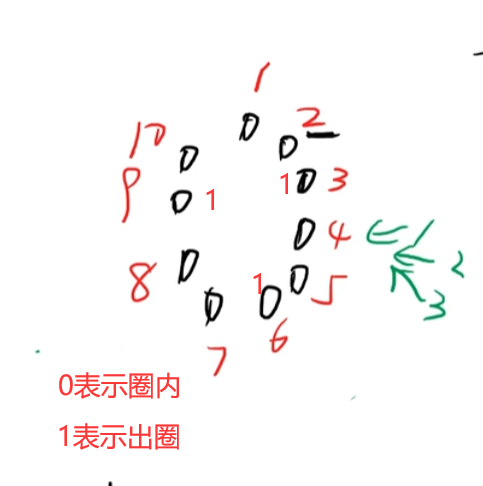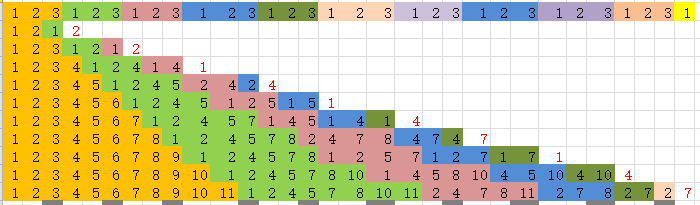• ## 约瑟夫问题c++

千次阅读 2017-10-15 16:54:22
约瑟夫问题c++


// ConsoleApp1.cpp : 定义控制台应用程序的入口点。
//

#include "stdafx.h"
#include<stdlib.h>
#include<stdio.h>
#include<malloc.h>
typedef struct List
{

int  data;
struct List *next;
}
展开全文• = n) //当还有人没出圈循环 { c++; //当前报数人的编号 //如果报数的人超过总人数，从第一个人开始报 if(c > n) { c=1; } if(a[c] == 0) //编号为c的人在圈里才报数 { k++; //当前编号为c的人报的数为k if(k == m) {...
#include <iostream>
using namespace std;
int main()
{
int a = {0}; //0表示在圈内
int n,m;
cout << "请输入人数，出圈数字 " << endl;
cin >> n >> m;   //n个人玩游戏，玩到m时出圈
int pop=0,c=0,k=0; //pop出圈
while(pop != n) //当还有人没出圈循环
{
c++;  //当前报数人的编号
//如果报数的人超过总人数，从第一个人开始报
if(c > n)
{
c=1;
}
if(a[c] == 0) //编号为c的人在圈里才报数
{
k++; //当前编号为c的人报的数为k
if(k == m)
{
a[c] = 1;
pop++;
cout << c << " ";
k=0;  //使下一个重新从1开始报数
}
}
}
return 0;
}展开全文• 约瑟夫问题c++代码，用链表编的 适合初学者~~~~~
• C++解决约瑟夫问题啊，解决约瑟夫问题u，C++
• 约瑟夫环（Josephus）问题是由古罗马的史学家约瑟夫（Josephus）提出的，其表述方式有多种，较典型的一种是： 有ｎ只猴子，按顺时针方向围成一圈选大王（编号从１到ｎ），从第１号开始报数，一直数到ｍ，数到ｍ的...
约瑟夫环（Josephus）问题是由古罗马的史学家约瑟夫（Josephus）提出的，其表述方式有多种，较典型的一种是： 有ｎ只猴子，按顺时针方向围成一圈选大王（编号从１到ｎ），从第１号开始报数，一直数到ｍ，数到ｍ的猴子退出圈外，剩下的猴子再接着从1 开始报数。就这样，直到圈内只剩下一只猴子时，这个猴子就是猴王，编程求输入ｎ，ｍ后，输出最后猴王的编号。
解法一：数组实现
根据输入的n值确定数组的长度，对数组中的n个元素依次编号i+1(0≤i<n)，然后这n个元素再进行从1到m的报数j(0≤j≤m)，每当报到m时(m==j)，将数组中对应元素的值置为0，并令j=0，总人数减1(cnt–)；该元素后面的一个元素重新从1开始报数(j++)。（由于是数组实现，难以及时将置为0的空间删除，所以在报数前需要检验该元素是否为0(a[i]!=0?）。 当总人数(cnt)减为1时退出while()循环，输出数组中值不为0的元素，即最后留下的元素序号。
#include <iostream>
using namespace std;

int main()
{
int n,m,cnt;
cout<<"n=";
cin>>n;
cout<<"m=";
cin>>m;
int a[n];
for(int i=0;i<n;i++)
a[i]=i+1;
cnt=n;
int j=0;
while(cnt>1)
{
for(int i=0;i<n;i++)
{
if(a[i]!=0)
j++;
if(j==m)
{
j=0;
a[i]=0;
cnt--;
}
}
}
for(int i=0;i<n;i++)
if(a[i]!=0)
cout<<a[i]<<endl;
return 0;
}

解法二：循环链表实现
创建一个由n个结点（含头结点）构成的循环链表，每个结点的数字域存放1~n的整型值。这n个结点进行1到m的报数，每当报到m时，删除对应的结点(delete temp)，并使n–，直到n减为1，退出循环，输出剩下的这个结点的数字域的值，即为该结点的序号。
#include<iostream>
using namespace std;

typedef struct SNode
{
int data;
SNode *next;
}SLNode;

main()
{
int ListJoseph(int n,int m);
int n,m;
cout<<"n=";
cin>>n;
cout<<"m=";
cin>>m;
ListJoseph(n,m);
return 0;
}

int ListJoseph(int n,int m)
{
int j=2;
if(n<2)
{
cout<<"总人数过少"<<endl;
return 0;
}
while(j<=n)
{
/*在链表末尾新增一个结点，并使新增结点的next指针指向头结点*/
q=new SLNode;
q->data=j;
p->next=q;
p=q;
j++;
}

int key=1;
while(n>1)
{
key++;
if(key==m)
{
/*当key==m时，删除对应的结点(p->next)*/
SLNode *temp;
temp=p->next;
delete temp;
p->next=p->next->next;
n--;
key=1;
}
p=p->next;
}
cout<<p->data<<endl;
return 1;
}

解法三：递归实现
在网上查到了用递归解决约瑟夫问题的代码（https://blog.csdn.net/m15682532244/article/details/78304165），代码很简短，但花了好几个小时仍不能完全理解，似乎理解了个大概，但自己无法说清具体是怎么回事。 我炮制的代码如下：
#include <iostream>
using namespace std;

int main()
{
int Joseph(int n,int m);
int n,m;
cout<<"n=";
cin>>n;
cout<<"m=";
cin>>m;
cout<<Joseph(n,m)<<endl;
return 0;
}

int Joseph(int n,int m)
{
if(n==2)
return((m%n)+1);
else
return((Joseph(n-1,m)-1+m)%n+1);
}

我令m=3，对2至11间的n值进行验证，代码是正确的，但其中的道理，是我所不能言说的，只将验证过程附在下面： （第一行的值是1,2,3的循环，表示下面每一行的报数情况。下面每行最后标红的数字，是最后留下的数字。）展开全文算法
• 经典约瑟夫问题 题目内容：有n只猴子，按顺时针方向围成一圈选大王（编号从1到n），从第1号开始报数，一直数到m，数到m的猴子退出圈外，剩下的猴子再接着从1开始报数。就这样，直到圈内只剩下一只猴子时，这个猴子...
经典约瑟夫问题
题目内容：有n只猴子，按顺时针方向围成一圈选大王（编号从1到n），从第1号开始报数，一直数到m，数到m的猴子退出圈外，剩下的猴子再接着从1开始报数。就这样，直到圈内只剩下一只猴子时，这个猴子就是猴王，编程求输入n，m后，输出最后猴王的编号。
输入格式:输入包含两个整数，第一个是n，第二个是m (0 < m,n <=300)。
输出格式：输出包含一行，即最后猴王的编号。
输入样例：18 2
输出样例：5
下面是Python版本，有注释帮助理解，C++同样的原理
n,m=input().split()
n=int(n)#输入n和m
m=int(m)
c=[]
for i in range(n):
c.append(0)
#初始化一个大小为n的数组，0代表此位置的猴子还没有被淘汰
n1=n
j=-1
#直到只剩一只猴子，游戏才结束
while n1>1:
n1-=1
#m次报数开始
for i in range(m):

temp=(j+1)%n
#如果下一个位置上的猴子还在话，就将j移动到这儿
if(c[temp]==0):
j+=1
else:
#否则就移动两个位置，但此位置的猴子也可能不在，所以用while来循环判断直到找到下一个猴子
j+=2
while(c[j%n]==1):
j+=1
#如果j大于n，再从头开始循环
if j>=n:
j=j%n
#找到猴子淘汰他
c[j]=1
#0就是最后一个猴子
for i in range(n):
if c[i]==0:
print(i+1)
break
C++版代码
#include <iostream>
#include<cstdio>
#include<algorithm>
#include <cstring>
#define MAX 210
using namespace  std;
int main(int argc, char const *argv[])
{   int n,m,n1;
scanf("%d%d",&n,&m);
int a[n];
memset(a,0,sizeof(a));
if (n==1)
{
printf("0" );
return 0;
}
int j=-1;
n1=n;

while(n1>1)
{   n1--;

for (int i = 0; i < m; ++i)
{
int temp=(j+1)%n;
if (a[temp]==0)
{
j++;
}
else{
j+=2;
while(a[j%n]==1)
{
j++;
}
}

if(j>=n)
j=j%n;
}
a[j]=1;
}
for (int i = 0; i < n; ++i)
{
if(a[i]==0)
{    printf("%d",i+1 );
break;
}
}

return 0;
}

展开全文C Python
• 用链表与循环完成约瑟夫问题 while (cin>>x>>y) { node *p, *q; //建立循环链表 for (i = 1; i ; i++) { if (i == 1) { list->head = new node; list->head->data = i; q = list->head; ...
• c++链表解决约瑟夫问题 n 个人围成一个圆圈，首先第1个人从1开始一个人一个人顺时针报数, 报到第m个人，令其出列。然后再从下一个人开始，从1顺时针报数，报到第m个人，再令其出列，…，如此下去, 直到圆圈中只剩...
• 约瑟夫问题，希望大佬可以帮帮我 有n人（编号分别为1到n号）围成一圈，从第s人开始报数，报到第m的人出列，然后从出列的下一人重新开始报数，报到第m的人又出列，...
• #include//c++中标准的输入和输出流 using namespace std;//使用标准的名空间std #include//因为后面要有list容器，所以要引用list的头文件 #include<stdlib.h>//system(“pause”)要用 void list1(int m, int...
• 简单易懂，用循环链表实现的约瑟夫问题，主要用于学校数据结构课程实验的参考
• ## 约瑟夫问题C++解析

千次阅读 2018-01-13 09:27:28
约瑟夫问题就是N个人围坐一圈，从1开始报数最大为M，当到M时重新从1开始。每次报数报到M的人便die，同时从他下一个人从新开始报数，最后只剩下1个人。 int main() { int n;//总人数 int m;//一次数m个人 int ...
• * Function : 约瑟夫问题 C++实现 * Created on : 2016年5月11日 * Author : beijiwei@qq.com * Copyright : 欢迎大家和我一起交流学习，转载请保持源文件的完整性。 任何单位和个人不经本人允许不得用于商业用途 * ...
• 1748:约瑟夫问题 查看 提交 统计 提问 总时间限制: 1000ms 内存限制: 65536kB 描述 约瑟夫问题：有ｎ只猴子，按顺时针方向围成一圈选大王（编号从１到ｎ），从第１号开始报数，一直数到ｍ，数到ｍ的猴子退出圈外，...算法
• 该程序用c++编写。约瑟夫问题可以用指针链表实现也可以用数组实现。这里提供一个用数组实现的，链表的可参考该算法实现
• ## 约瑟夫问题C++求解

千次阅读 2013-10-04 23:40:23
#include using namespace std; struct child { int id; child *nextChild;...总之约瑟夫就会一个单向循环链表的问题 注意建表的细节并且在遍历的时候注意保存前面的节点 还有清除节点的过程
• #include #include #include using namespace std; int main() {  int N,M;  cin>>N>>M;  vectorv;  int before=0; for(int i=1;i while(!v.empty()) ... before=(before+M-1)%v.
• 总时间限制:1000ms内存限制:65536kB描述约瑟夫问题：有ｎ只猴子，按顺时针方向围成一圈选大王（编号从１到ｎ），从第１号开始报数，一直数到ｍ，数到ｍ的猴子退出圈外，剩下的猴子再接着从1开始报数。就这样，直到圈...
• ## 约瑟夫问题C++实现

千次阅读 2008-10-31 09:59:00
//*************************** //** Josephus问题解法1 ** //** jose1.cpp ** //*************************** #include void main()input
• 假设有十个人围成一圈，开始数数，数到8的人离开，直到剩余最后一个人为止。用程序数组（非指针）来演示这个过程#includeusing namespace std;void main(){int counter=0;int array={1,1,1,1,1,1,1,1,1,1};...
• 约瑟夫C++ 约瑟夫C++ 约瑟夫C++
• 约瑟夫问题C++解决方案，挺好用的.约瑟夫问题C++解决方案，挺好用的.
• 约瑟夫问题 C++ 学生用 数据结构作业实用代码...

# 约瑟夫问题c++c++ 订阅